We think you are located in United States. Is this correct?

# 5.4 Exponential functions

## 5.4 Exponential functions (EMBGS)

### Revision (EMBGT)

#### Functions of the form $$y=a{b}^{x}+q$$

Functions of the general form $$y=a{b}^{x}+q$$, for $$b>0$$, are called exponential functions, where $$a$$, $$b$$ and $$q$$ are constants.

The effects of $$a$$, $$b$$ and $$q$$ on $$f(x) = ab^x + q$$:

• The effect of $$q$$ on vertical shift

• For $$q>0$$, $$f(x)$$ is shifted vertically upwards by $$q$$ units.

• For $$q<0$$, $$f(x)$$ is shifted vertically downwards by $$q$$ units.

• The horizontal asymptote is the line $$y = q$$.

• The effect of $$a$$ on shape

• For $$a>0$$, $$f(x)$$ is increasing.

• For $$a<0$$, $$f(x)$$ is decreasing. The graph is reflected about the horizontal asymptote.

• The effect of $$b$$ on direction

Assuming $$a > 0$$:

• If $$b > 1$$, $$f(x)$$ is an increasing function.
• If $$0 < b < 1$$, $$f(x)$$ is a decreasing function.
• If $$b \leq 0$$, $$f(x)$$ is not defined.
 $$b>1$$ $$a<0$$ $$a>0$$ $$q>0$$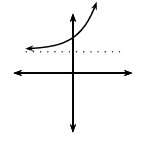$$q<0$$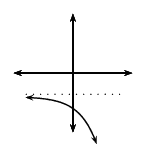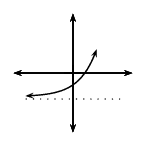$$00$$ $$q>0$$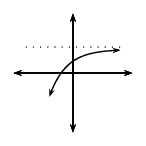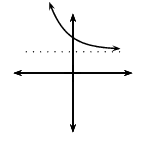$$q<0$$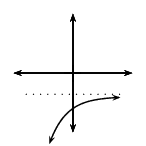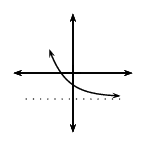## Revision

Textbook Exercise 5.15

On separate axes, accurately draw each of the following functions:

• Use tables of values if necessary.
• Use graph paper if available.

$$y_1 = 3^x$$$$y_2 = -2 \times 3^x$$$$y_3 = 2 \times 3^x + 1$$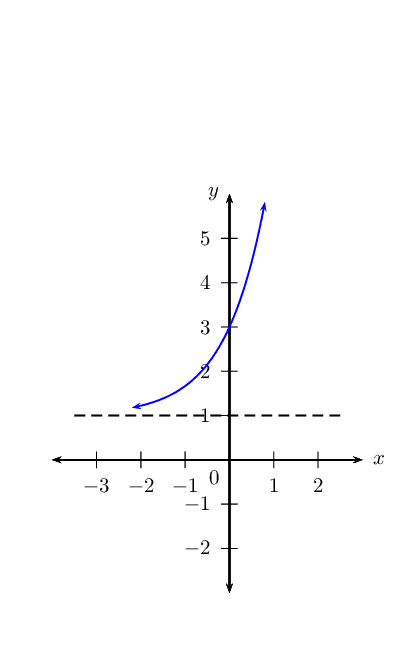$$y_4 = 3^x - 2$$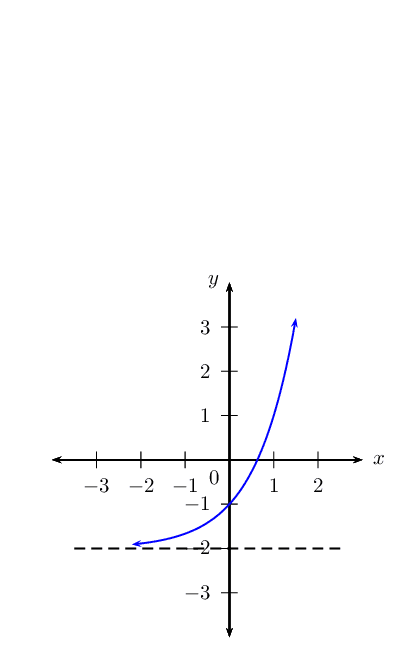Use your sketches of the functions given above to complete the following table (the first column has been completed as an example):

 $$y_1$$ $$y_2$$ $$y_3$$ $$y_4$$ value of $$q$$ $$q = 0$$ effect of $$q$$ no vertical shift value of $$a$$ $$a = 1$$ effect of $$a$$ increasing asymptote $$x$$-axis, $$y = 0$$ domain $$\{x: x \in \mathbb{R} \}$$ range $$\{y: y \in \mathbb{R}, y > 0 \}$$
 $$y_1$$ $$y_2$$ $$y_3$$ $$y_4$$ value of $$q$$ $$q = 0$$ $$q = 0$$ $$q = 1$$ $$q = 2$$ effect of $$q$$ no vertical shift no vertical shift shift $$1$$ unit up shift $$2$$ units down value of $$a$$ $$a = 1$$ $$a = -2$$ $$a = 2$$ $$a = 1$$ effect of $$a$$ increasing decreasing increasing increasing asymptote $$x$$-axis, $$y = 0$$ $$x$$-axis, $$y = 0$$ $$y = 1$$ $$y = -2$$ domain $$\{x: x \in \mathbb{R} \}$$ $$\{x: x \in \mathbb{R} \}$$ $$\{x: x \in \mathbb{R} \}$$ $$\{x: x \in \mathbb{R} \}$$ range $$\{y: y \in \mathbb{R}, y > 0 \}$$ $$\{y: y \in \mathbb{R}, y < 0 \}$$ $$\{y: y \in \mathbb{R}, y > 1 \}$$ $$\{y: y \in \mathbb{R}, y > -2 \}$$

### Functions of the form $$y=a{b}^{\left(x+p\right)}+q$$ (EMBGV)

We now consider exponential functions of the form $$y=a{b}^{\left(x+p\right)}+q$$ and the effects of parameter $$p$$.

## The effects of $$a$$, $$p$$ and $$q$$ on an exponential graph

1. On the same system of axes, plot the following graphs:

1. $$y_1 = 2^x$$
2. $$y_2 = 2^{(x - 2)}$$
3. $$y_3 = 2^{(x - 1)}$$
4. $$y_4 = 2^{(x + 1)}$$
5. $$y_5 = 2^{(x + 2)}$$

Use your sketches of the functions above to complete the following table:

 $$y_1$$ $$y_2$$ $$y_3$$ $$y_4$$ $$y_5$$ intercept(s) asymptote domain range effect of $$p$$
2. On the same system of axes, plot the following graphs:

1. $$y_1 = 2^{(x - 1)} + 2$$
2. $$y_2 = 3 \times 2^{(x - 1)} + 2$$
3. $$y_3 = \frac{1}{2} \times 2^{(x - 1)} + 2$$
4. $$y_4 = 0 \times 2^{(x - 1)} + 2$$
5. $$y_5 = -3 \times 2^{(x - 1)} + 2$$

Use your sketches of the functions above to complete the following table:

 $$y_1$$ $$y_2$$ $$y_3$$ $$y_4$$ $$y_5$$ intercept(s) asymptotes domain range effect of $$a$$

The effect of the parameters on $$y = ab^{x + p} + q$$

The effect of $$p$$ is a horizontal shift because all points are moved the same distance in the same direction (the entire graph slides to the left or to the right).

• For $$p>0$$, the graph is shifted to the left by $$p$$ units.

• For $$p<0$$, the graph is shifted to the right by $$p$$ units.

The effect of $$q$$ is a vertical shift. The value of $$q$$ also affects the horizontal asymptotes, the line $$y = q$$.

The value of $$a$$ affects the shape of the graph and its position relative to the horizontal asymptote.

• For $$a>0$$, the graph lies above the horizontal asymptote, $$y = q$$.

• For $$a<0$$, the graph lies below the horizontal asymptote, $$y = q$$.

 $$p>0$$ $$p<0$$ $$a<0$$ $$a>0$$ $$a<0$$ $$a>0$$ $$q>0$$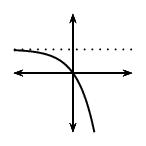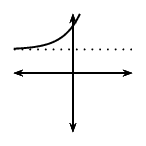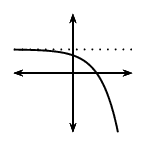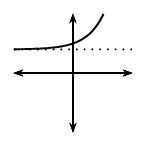$$q<0$$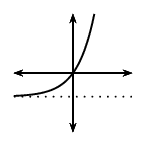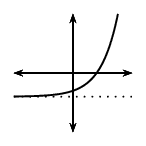#### Discovering the characteristics

For functions of the general form: $$f(x) = y = ab^{(x+p)} + q$$:

Domain and range

The domain is $$\left\{x:x\in ℝ\right\}$$ because there is no value of $$x$$ for which $$f(x)$$ is undefined.

The range of $$f(x)$$ depends on whether the value for $$a$$ is positive or negative.

If $$a>0$$ we have: \begin{align*} {b}^{\left(x+p\right)} & > 0 \\ a {b}^{\left(x+p\right)} & > 0 \\ a {b}^{\left(x+p\right)} + q & > q \\ f(x) & > q \end{align*} The range is therefore $$\{ y: y > q, y \in \mathbb{R} \}$$.

Similarly, if $$a < 0$$, the range is $$\{ y: y < q, y \in \mathbb{R} \}$$.

## Worked example 14: Domain and range

State the domain and range for $$g(x) = 5 \times 3^{(x+1)} - 1$$.

### Determine the domain

The domain is $$\{x: x \in \mathbb{R} \}$$ because there is no value of $$x$$ for which $$g(x)$$ is undefined.

### Determine the range

The range of $$g(x)$$ can be calculated from: \begin{align*} 3^{(x+1)} & > 0\\ 5 \times 3^{(x+1)} & > 0\\ 5 \times 3^{(x+1)} - 1 & > -1\\ \therefore g(x) & > -1 \end{align*} Therefore the range is $$\{g(x): g(x) > -1 \}$$ or in interval notation $$(-1; \infty)$$.

## Domain and range

Textbook Exercise 5.16

Give the domain and range for each of the following functions:

$$y = \left( \frac{3}{2} \right)^{(x + 3)}$$

\begin{align*} \text{Domain: } & \left \{ x: x \in \mathbb{R} \right \} \\ \text{Range: } & \left \{ y: y > 0, y\in \mathbb{R} \right \} \end{align*}

$$f(x) = -5^{(x - 2)} + 1$$

\begin{align*} \text{Domain: } & \left \{ x: x \in \mathbb{R} \right \} \\ \text{Range: } & \left \{ y: y < 1, y\in \mathbb{R} \right \} \end{align*}

$$y + 3 = 2^{(x + 1)}$$

\begin{align*} y + 3 &= 2^{(x + 1)} \\ y &= 2^{(x + 1)} - 3 \\ \text{Domain: } & \left \{ x: x \in \mathbb{R} \right \} \\ \text{Range: } & \left \{ y: y > -3, y\in \mathbb{R} \right \} \end{align*}

$$y = n + 3^{(x - m)}$$

\begin{align*} y &= n + 3^{(x - m)} \\ y &= 3^{(x - m)} + n \\ \text{Domain: } & \left \{ x: x \in \mathbb{R} \right \} \\ \text{Range: } & \left \{ y: y > n, y\in \mathbb{R} \right \} \end{align*}

$$\frac{y}{2} = 3^{(x - 1)} - 1$$

\begin{align*} \frac{y}{2} &= 3^{(x - 1)} - 1 \\ y &= 2 \times 3^{(x - 1)} - 2 \\ \text{Domain: } & \left \{ x: x \in \mathbb{R} \right \} \\ \text{Range: } & \left \{ y: y > 2, y\in \mathbb{R} \right \} \end{align*}

Intercepts

The $$y$$-intercept:

To calculate the $$y$$-intercept we let $$x=0$$. For example, the $$y$$-intercept of $$g(x) = 3 \times 2^{(x + 1)} + 2$$ is determined by setting $$x=0$$: \begin{align*} g(0) &= 3 \times 2^{(0 + 1)} + 2 \\ &= 3 \times 2 + 2\\ &= 8 \end{align*} This gives the point $$(0;8)$$.

The $$x$$-intercept:

To calculate the $$x$$-intercept we let $$y=0$$. For example, the $$x$$-intercept of $$g(x) = 3 \times 2^{(x + 1)} + 2$$ is determined by setting $$y=0$$: \begin{align*} 0 &= 3 \times 2^{(x + 1)} + 2 \\ -2 &= 3 \times 2^{(x + 1)} \\ -\frac{2}{3} &= 2^{(x + 1)} \end{align*} which has no real solutions. Therefore, the graph of $$g(x)$$ lies above the $$x$$-axis and does not have any $$x$$-intercepts.

## Intercepts

Textbook Exercise 5.17

Determine the $$x$$- and $$y$$-intercepts for each of the following functions:

$$f(x) = 2^{(x + 1)} - 8$$

\begin{align*} \text{For } x=0 \quad y &= 2^{(0 + 1)} - 8 \\ &= 2 - 8 \\ &= -6 \\ \therefore & (0;-6) \\ \text{For } y=0 \quad 0 &= 2^{(x + 1)} - 8 \\ 2^3 &= 2^{(x + 1)} \\ \therefore 3 &= x + 1 \\ \therefore 2 &= x \\ \therefore & (2;0) \end{align*}

$$y = 2 \times 3^{(x - 1)} - \text{18}$$

\begin{align*} \text{For } x=0 \quad y &= 2 \times 3^{(0 - 1)} - 18 \\ &= \frac{2}{3} -18 \\ &= -17\frac{1}{3} \\ \therefore & (0;-17\frac{1}{3}) \\ \text{For } y=0 \quad 0 &= 2 \times 3^{(x - 1)} - 18 \\ 18 &= 2 \times 3^{(x - 1)} \\ 9 &= 3^{(x - 1)} \\ 3^2 &= 3^{(x - 1)} \\ \therefore 2 &= x - 1 \\ \therefore 3 &= x \\ \therefore & (3;0) \end{align*}

$$y + 5^{(x + 2)} = 5$$

\begin{align*} y + 5^{(x + 2)} &= 5 \\ y &= -5^{(x + 2)} + 5 \\ \text{For } x=0 \quad y &= -5^{(0 + 2)} + 5 \\ &= -25 + 5 \\ &= -20 \\ \therefore & (0;-20) \\ \text{For } y=0 \quad 0 &= -5^{(x + 2)} + 5 \\ 5^{(x + 2)} &= 5\\ \therefore x + 2 &= 1 \\ \therefore x &= -1 \\ \therefore & (-1;0) \end{align*}

$$y = \frac{1}{2} \left( \frac{3}{2} \right)^{(x + 3)} - \text{0,75}$$

\begin{align*} y &= \frac{1}{2} \left( \frac{3}{2} \right)^{(x + 3)} - \text{0,75} \\ y &= \frac{1}{2} \left( \frac{3}{2} \right)^{(x + 3)} - \frac{3}{4} \\ \text{For } x=0 \quad y &= \frac{1}{2} \left( \frac{3}{2} \right)^{(0 + 3)} - \frac{3}{4} \\ &= \frac{1}{2} \left( \frac{3}{2} \right)^{3} - \frac{3}{4} \\ &= \frac{1}{2} \left( \frac{27}{8} \right)- \frac{3}{4} \\ &= \frac{27}{16} - \frac{3}{4} \\ &= \frac{15}{16} \\ \therefore & (0;\frac{15}{16}) \\ \text{For } y=0 \quad 0 &= \frac{1}{2} \left( \frac{3}{2} \right)^{(x + 3)} - \frac{3}{4} \\ \frac{3}{4} &= \frac{1}{2} \left( \frac{3}{2} \right)^{(x + 3)} \\ \frac{3}{2} &= \left( \frac{3}{2} \right)^{(x + 3)} \\ \therefore 1 &= x + 3 \\ \therefore -2 &= x \\ \therefore & (-2;0) \end{align*}

Asymptote

Exponential functions of the form $$y = ab^{(x+p)} + q$$ have a horizontal asymptote, the line $$y = q$$.

## Worked example 15: Asymptote

Determine the asymptote for $$y = 5 \times 3^{(x+1)} - 1$$.

### Determine the asymptote

The asymptote of $$g(x)$$ can be calculated as: \begin{align*} 3^{(x+1)} & \ne 0\\ 5 \times 3^{(x+1)} & \ne 0\\ 5 \times 3^{(x+1)} - 1 & \ne -1\\ \therefore y & \ne -1 \end{align*} Therefore the asymptote is the line $$y = -1$$.

## Asymptote

Textbook Exercise 5.18

Give the asymptote for each of the following functions:

$$y = -5^{(x + 1)}$$

\begin{align*} y &= -5^{(x + 1)} \\ \text{Horizontal asymptote: } \quad y &= 0 \end{align*}

$$y = 3^{(x - 2)} + 1$$

\begin{align*} y &= 3^{(x - 2)} + 1 \\ \text{Horizontal asymptote: } \quad y &= 1 \end{align*}

$$\left( \frac{3y}{2} \right) = 5^{(x + 3)} - 1$$

\begin{align*} \left( \frac{3y}{2} \right) &= 5^{(x + 3)} - 1 \\ 3y &= 2 \times 5^{(x + 3)} - 2 \\ y &= \frac{2}{3} \times 5^{(x + 3)} - \frac{2}{3} \\ \text{Horizontal asymptote: } \quad y &= -\frac{2}{3} \end{align*}

$$y = 7^{(x + 1)} - 2$$

\begin{align*} y &= 7^{(x + 1)} - 2 \\ \text{Horizontal asymptote: } \quad y &= -2 \end{align*}

$$\frac{y}{2} + 1 = 3^{(x + 2)}$$

\begin{align*} \frac{y}{2} + 1 &= 3^{(x + 2)} \\ \frac{y}{2} &= 3^{(x + 2)} - 1 \\ y &= 2 \times 3^{(x + 2)} - 2 \\ \text{Horizontal asymptote: } \quad y &= -2 \end{align*}

#### Sketching graphs of the form $$f(x)=a{b}^{\left(x+p\right)}+q$$

In order to sketch graphs of functions of the form, $$f(x)=a{b}^{\left(x+p\right)}+q$$, we need to determine five characteristics:

• shape

• $$y$$-intercept

• $$x$$-intercept

• asymptote

• domain and range

## Worked example 16: Sketching an exponential graph

Sketch the graph of $$2y = \text{10} \times 2^{(x+1)} - 5$$.

Mark the intercept(s) and asymptote. State the domain and range of the function.

### Examine the equation of the form $$y = ab^{(x + p)} + q$$

We notice that $$a > 0$$ and $$b > 1$$, therefore the function is increasing.

### Determine the $$y$$-intercept

The $$y$$-intercept is obtained by letting $$x = 0$$: \begin{align*} 2y &= \text{10} \times 2^{(0+1)} - 5\\ &= \text{10} \times 2 - 5\\ &= \text{15}\\ \therefore y &= 7\frac{1}{2} \end{align*} This gives the point $$(0;7\frac{1}{2})$$.

### Determine the $$x$$-intercept

The $$x$$-intercept is obtained by letting $$y = 0$$: \begin{align*} 0 &= \text{10} \times 2^{(x+1)} - 5\\ 5 &= \text{10} \times 2^{(x+1)} \\ \frac{1}{2} &= 2^{(x+1)}\\ 2^{-1} &= 2^{(x+1)}\\ \therefore -1 &= x + 1 \quad \text{(same base)}\\ -2 &= x \end{align*} This gives the point $$(-2;0)$$.

### Determine the asymptote

The horizontal asymptote is the line $$y = -\frac{5}{2}$$.

### Plot the points and sketch the graph### State the domain and range

Domain: $$\{ x: x \in \mathbb{R} \}$$

Range: $$\{ y: y > -\frac{5}{2}, y \in \mathbb{R} \}$$

Finding the equation of an exponential function from the graph

## Worked example 17: Finding the equation of an exponential function from the graph

Use the given graph of $$y = -2 \times 3^{(x + p)} + q$$ to determine the values of $$p$$ and $$q$$.### Examine the equation of the form $$y = ab^{(x + p)} + q$$

From the graph we see that the function is decreasing. We also note that $$a = -2$$ and $$b = 3$$.

We need to solve for $$p$$ and $$q$$.

### Use the asymptote to determine $$q$$

The horizontal asymptote $$y = 6$$ is given, therefore we know that $$q = 6$$. $y = -2 \times 3^{(x + p)} + 6$

### Use the $$x$$-intercept to determine $$p$$

Substitute $$(2;0)$$ into the equation and solve for $$p$$: \begin{align*} y &= -2 \times 3^{(x + p)} + 6 \\ 0 &= -2 \times 3^{(2 + p)} + 6 \\ -6 &= -2 \times 3^{(2 + p)} \\ 3 &= 3^{(2 + p)} \\ \therefore 1 &= 2 + p \quad \text{(same base)}\\ \therefore p &= -1 \end{align*}

$y = -2 \times 3^{(x - 1)} + 6$

## Mixed exercises

Textbook Exercise 5.19

Given the graph of the hyperbola of the form $$h(x) = \frac{k}{x}$$, $$x < 0$$, which passes though the point $$A(-\frac{1}{2}; -6)$$.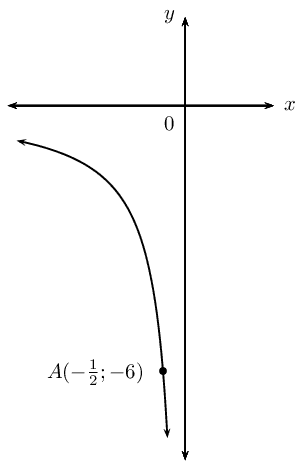Show that $$k=3$$.

\begin{align*} y &=\frac{k}{x} \\ \text{Subst. } (-\frac{1}{2}; -6) \qquad -6 &=\frac{k}{-\frac{1}{2}} \\ -6 \times -\frac{1}{2} &= k \\ \therefore k &=3 \\ \therefore h(x) &= \frac{3}{x} \end{align*}

Write down the equation for the new function formed if $$h(x)$$:

is shifted $$\text{3}$$ units vertically upwards

\begin{align*} h(x) &= \frac{3}{x} \\ \therefore y &\Rightarrow y - 3 \\ y - 3 &=\frac{3}{x} \\ y &=\frac{3}{x}+3 \end{align*}

is shifted to the right by $$\text{3}$$ units

\begin{align*} h(x) &= \frac{3}{x} \\ \therefore x &\Rightarrow x - 3 \\ y &=\frac{3}{x -3} \end{align*}

is reflected about the $$y$$-axis

\begin{align*} h(x) &= \frac{3}{x} \\ \therefore x &\Rightarrow -x \\ y &=\frac{3}{x -3} \end{align*}

is shifted so that the asymptotes are $$x = 0$$ and $$y = -\frac{1}{4}$$

\begin{align*} h(x) &= \frac{3}{x} \\ \therefore p=0 &\text{ and } q = -\frac{1}{4} \\ y &=\frac{3}{x} -\frac{1}{4} \end{align*}

is shifted upwards to pass through the point $$(-1;1)$$

\begin{align*} h(x) &= \frac{3}{x} \\ \therefore y &\Rightarrow y + m \\ y &=\frac{3}{x} + m \\ \text{Subst.} (-1;1) \qquad 1 &= \frac{3}{-1} + m \\ 1 + 3 &= + m \\ \therefore m &= 4 \\ \therefore y &=\frac{3}{x} + 4 \end{align*}

is shifted to the left by $$\text{2}$$ units and $$\text{1}$$ unit vertically downwards (for $$x < 0$$)

\begin{align*} h(x) &= \frac{3}{x} \\ \therefore x &\Rightarrow x + 2 \\ \therefore y &\Rightarrow y + 1 \\ y + 1 &=\frac{3}{x + 2} \\ \therefore y &=\frac{3}{x + 2} - 1 \end{align*}

Given the graphs of $$f(x) = a(x+p)^2$$ and $$g(x) = \frac{a}{x}$$.

The axis of symmetry for $$f(x)$$ is $$x = -1$$ and $$f(x)$$ and $$g(x)$$ intersect at point $$M$$. The line $$y = 2$$ also passes through $$M$$.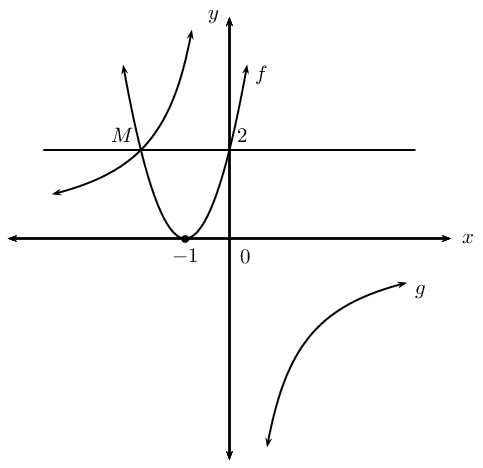Determine:

the coordinates of $$M$$

$$f(x)$$ is symmetrical about the line $$x = -1$$, therefore $$M(-2;2)$$.

the equation of $$g(x)$$

\begin{align*} g(x) &= \frac{a}{x} \\ \text{Subst. } M(-2;2) \qquad 2 &= \frac{a}{-2} \\ \therefore a &= -4 \\ \therefore g(x)&=\frac{-4}{x} \end{align*}

the equation of $$f(x)$$

\begin{align*} f(x) &= a(x + p)^2 + q \\ \text{No vertical shift } \therefore q &= 0 \\ \text{Axis of symmetry } x = -1 \qquad \therefore f(x) &= a(x + 1)^2 \\ \text{Subst. } M(-2;2) \qquad 2 &= a(-2 + 1)^2 \\ 2 &= a(-1)^2 \\ \therefore a &= 2 \\ \therefore f(x) &= 2(x + 1)^2 \end{align*}

the values for which $$f(x) < g(x)$$

$$- 2 < x < 0$$

the range of $$f(x)$$

$$\text{Range: }\left \{ y: y\in \mathbb{R}, y \geq 0 \right \}$$

On the same system of axes, sketch:

the graphs of $$k(x) = 2(x + \frac{1}{2})^2 - 4\frac{1}{2}$$ and $$h(x) = 2^{(x + \frac{1}{2})}$$. Determine all intercepts, turning point(s) and asymptotes.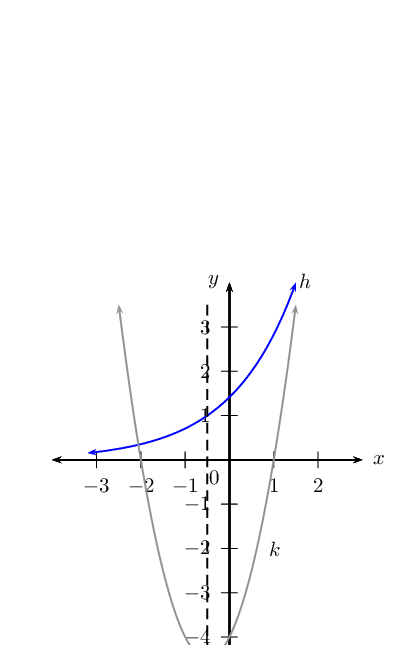the reflection of $$h(x)$$ about the $$x$$-axis. Label this function as $$j(x)$$.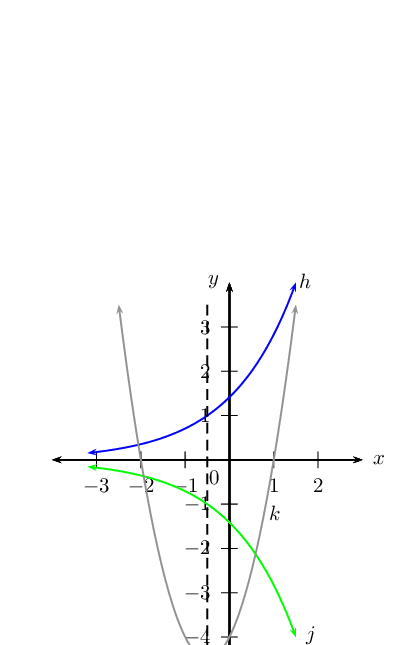Sketch the graph of $$y = ax^2 + bx + c$$ for:

$$a < 0$$, $$b > 0$$, $$b^2 < 4ac$$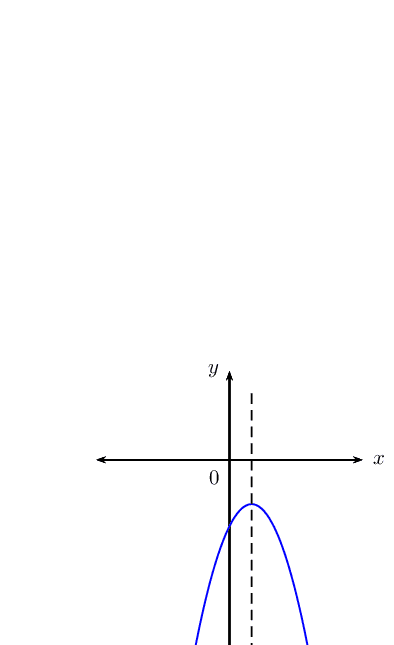$$a > 0$$, $$b > 0$$, one root $$=0$$On separate systems of axes, sketch the graphs:

$$y = \frac{2}{x - 2}$$

$$y = \frac{2}{x} - 2$$

$$y = -2^{(x - 2)}$$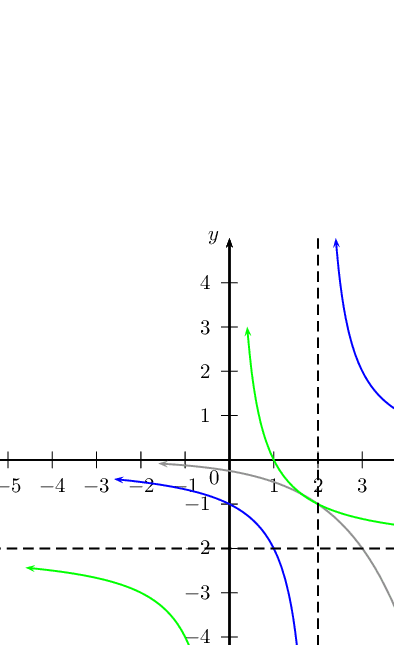For the diagrams shown below, determine:

• the equations of the functions; $$f(x) = a(x + p)^2 + q$$, $$g(x)=ax^2 + q$$, $$h(x) = \frac{a}{x}, x < 0$$ and $$k(x)=b^x + q$$
• the axes of symmetry of each function
• the domain and range of each function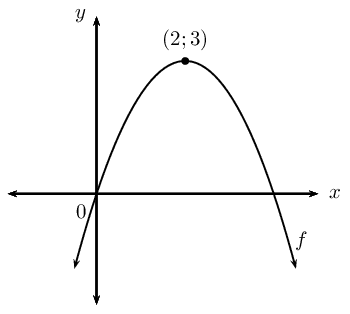\begin{align*} f(x) &= a(x + p)^2 + q \\ \text{From turning point: } p= -2 &\text{ and } q = 3 \\ \therefore f(x) &= a(x - 2 )^2 + 3 \\ \text{Subst. } (0;0) \qquad 0 &= a(0 - 2)^2 + 3 \\ -3 &= 4a \\ \therefore a &= -\frac{3}{4} \\ f(x) &= -\frac{3}{4}(x - 2)^2 + 3 \\ \text{Axes of symmetry: } x &= 2 \\ \text{Domain: } & \left \{ x:x \in \mathbb{R} \right \} \\ \text{Range: } & \left \{ y:y \in \mathbb{R}, y \leq 3 \right \} \end{align*}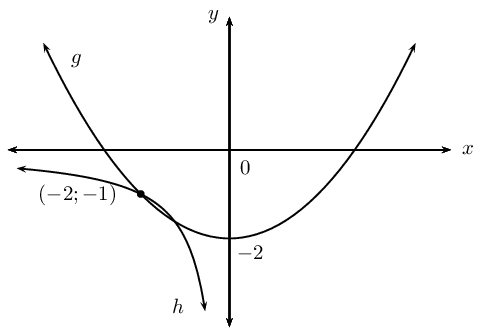\begin{align*} g(x) &= ax^2 + q \\ \text{From turning point: } p= 0 &\text{ and } q = -2 \\ \therefore g(x) &= a(x)^2 - 2 \\ \text{Subst. } (-2;-1) \qquad -1 &= a(-2 )^2 - 2\\ -1 &= 4a - 2\\ 1 &= 4a - 2\\ \therefore a &= \frac{1}{4} \\ g(x) &= \frac{1}{4}x^2 -2 \\ \text{Axes of symmetry: } x &= 0 \\ \text{Domain: } & \left \{ x:x \in \mathbb{R} \right \} \\ \text{Range: } & \left \{ y:y \in \mathbb{R}, y \geq -2 \right \} \\ h(x) &= \frac{a}{x + p} + q \\ \text{From graph: } p= 0 &\text{ and } q = 0 \\ h(x) &= \frac{a}{x} \\ \text{Subst. } (-2;-1) \qquad -1 &=\frac{a}{-2} \\ 2 &= a \\ \therefore h(x) &= \frac{2}{x} \\ \text{Axes of symmetry: } y &= x \\ \text{Domain: } &\left \{ x:x \in \mathbb{R}, x < 0 \right \} \\ \text{Range: } & \left \{ y:y \in \mathbb{R}, y < 0 \right \} \end{align*}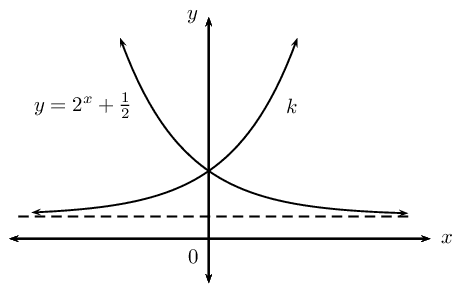\begin{align*} y &= 2^{x} + \frac{1}{2} \\ \text{Reflect about } x = 0 \qquad \therefore x &\\Rightarrow -x \\ \therefore k(x) &= 2^{-x} + \frac{1}{2} \\ &= \left( \frac{1}{2} \right)^{x} + \frac{1}{2} \\ \text{Domain: } & \left \{ x:x \in \mathbb{R} \right \} \\ \text{Range: } & \left \{ y:y \in \mathbb{R}, y > \frac{1}{2} \right \} \end{align*}

Given the graph of the function $$Q(x) = a^x$$.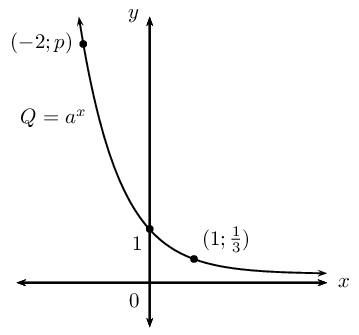Show that $$a = \frac{1}{3}$$.

\begin{align*} y&=a^x \\ \text{Subst.} \left( 1;\frac{1}{3} \right) \qquad \frac{1}{3} &=a^{1} \\ \therefore a &= \frac{1}{3} \end{align*}

Find the value of $$p$$ if the point $$(-2;p)$$ is on $$Q$$.

\begin{align*} Q(x) &= \left( \frac{1}{3} \right)^x \\ \text{Subst.} \left( -2;p \right) p &= \left( \frac{1}{3} \right)^{-2} \\ p &= 9 \end{align*}

Calculate the average gradient of the curve between $$x = -2$$ and $$x = 1$$.

\begin{align*} \text{Average gradient} &= \frac{\left ( \frac{1}{3} \right )^{-2} - \left ( \frac{1}{3} \right )^1}{-2-(1)} \\ &= \frac{9-\frac{1}{3}}{-3} \\ &=\frac{8\frac{2}{3}}{-3} \\ &= \frac{-26}{9} \\ &= -2\frac{8}{9} \end{align*}

Determine the equation of the new function formed if $$Q$$ is shifted $$\text{2}$$ units vertically downwards and $$\text{2}$$ units to the left.

\begin{align*} Q(x) &= \left( \frac{1}{3} \right)^x \\ \therefore x & \Rightarrow x + 2 \\ \therefore y & \Rightarrow y + 2 \\ y + 2 &= \left( \frac{1}{3} \right)^{x + 2} \\ y &= \left( \frac{1}{3} \right)^{x + 2} -2 \end{align*}

Find the equation for each of the functions shown below:

$$f(x) = 2^x + q$$

$$g(x) = mx + c$$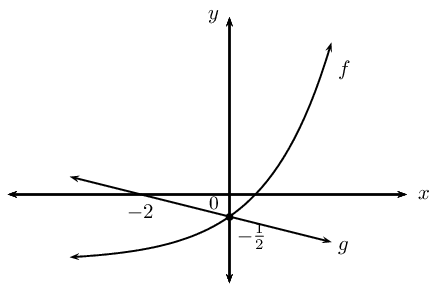\begin{align*} f(x) &= 2^x + q \\ \text{Subst. } (0; -\frac{1}{2}) \quad -\frac{1}{2} &= 2^{0} + q \\ -\frac{1}{2} &= 1 + q \\ \therefore q &= -\frac{3}{2} \\ \therefore f(x) &=2^x-\frac{3}{2} \\ g(x) &= mx +c \\ \text{Subst. } (0; -\frac{1}{2}) \quad -\frac{1}{2} &= m(0) + c \\ \therefore c &= -\frac{1}{2} \\ g(x) &= mx -\frac{1}{2} \\ \text{Subst. } (-2;0) \quad 0 &= m(-2) -\frac{1}{2} \\ \frac{1}{2} &= -2m \\ \therefore m &= -\frac{1}{4} \\ \therefore g(x) &= -\frac{1}{4}x-\frac{1}{2} \end{align*}

$$h(x) = \frac{k}{x + p} + q$$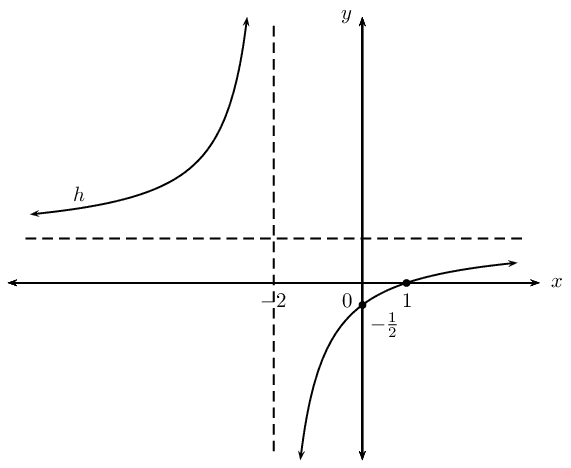\begin{align*} h(x) &= \frac{k}{x + p} + q \\ \text{From graph: } \quad p = -2 \\ h(x) &= \frac{k}{x + 2} + q \\ \text{Subst. } (0; -\frac{1}{2}) \quad -\frac{1}{2} &= \frac{k}{2} + q \\ -1 &= k + 2q \ldots (1)\\ \text{Subst. } (1;0) \quad 0 &= \frac{k}{1 + 2} + q \\ 0 &= k + 3q \ldots (2)\\ (2) - (1): \qquad 1 &= 0 + q \\ \therefore q &= 1 \\ \text{and } k &= -3\\ \therefore h(x) &= -\frac{3}{x + 2} + 1 \end{align*}

Given: the graph of $$k(x) = -x^2 + 3x + \text{10}$$ with turning point at $$D$$. The graph of the straight line $$h(x) = mx + c$$ passing through points $$B$$ and $$C$$ is also shown.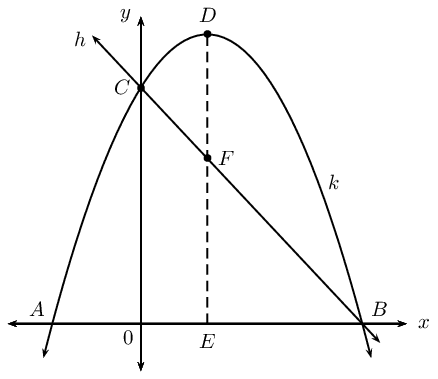Determine:

the lengths $$AO$$, $$OB$$, $$OC$$ and $$DE$$

\begin{align*} y &=-x^2+3x+10 \\ \text{Let } y &= 0 \\ 0 &=-x^2+3x+10 \\ &= x^2-3x-10\\ &= (x-5)(x+2)\\ \therefore x=5 &\text{ or }x =-2 \\ \therefore AO &= \text{2}\text{ units} \\ \therefore BO &=\text{5}\text{ units} \\ CO&=\text{10}\text{ units}\\ \text{Axes of symmetry: } x &=-\frac{5 - 2}{2} \\ &=\frac{3}{2} \\ \text{Subst. } x &= \frac{3}{2} \\ y &=-\left ( \frac{3}{2} \right )^2+3\frac{3}{2}+10 \\ &=12\frac{1}{4} \\ \therefore DE &= \text{12,25}\text{ units} \end{align*}

the equation of $$DE$$

$$DE = 12\frac{1}{4}$$

the equation of $$h(x)$$

\begin{align*} h(x) & = mx + c \\ h(x) & = mx + 10 \\ \text{Subst. } (5;0) \qquad 0 &= m(5) + 10 \\ -10 &= 5m \\ \therefore m &= -2 \\ \therefore h(x) &=-2x+10 \end{align*}

the $$x$$-values for which $$k(x) < 0$$

$$\left \{ x: x\in \mathbb{R}, x < -2 \text{ and } x > 5 \right \}$$

the $$x$$-values for which $$k(x) \geq h(x)$$

$$\left \{ x: x\in \mathbb{R}, 0 \leq x \leq 5 \right \}$$

the length of $$DF$$

\begin{align*} \text{At } x = \frac{3}{2} k(x) &= 12\frac{1}{4} \\ \text{At } x = \frac{3}{2} h(x) &= -2 \left( \frac{3}{2} \right) + 10 \\ &= -3 + 10 \\ &= 7\\ \therefore DF &= 12\frac{1}{4} - 7 \\ &= 12\frac{1}{4} - 7 \\ &= \text{5,25}\text{ units} \end{align*}

Trigonometric functions are examined in PAPER 2.